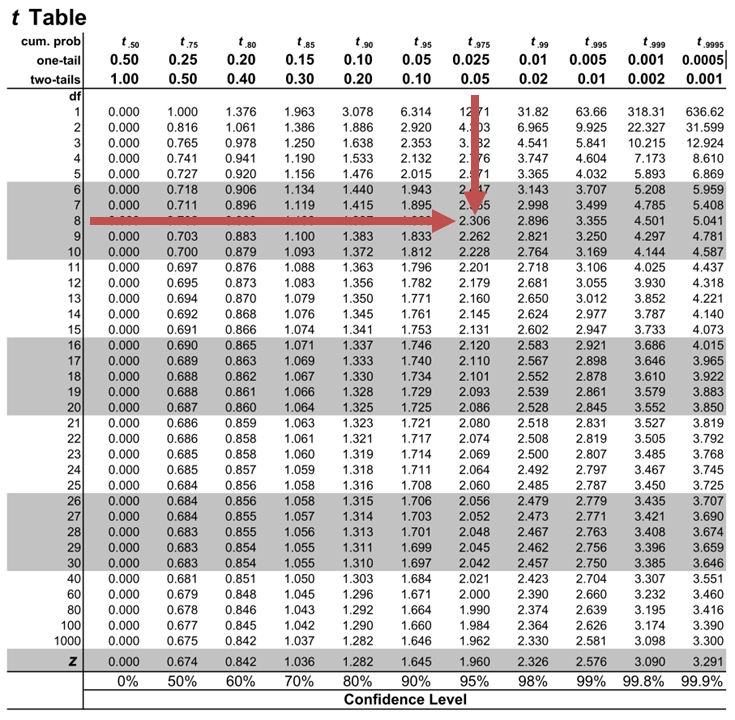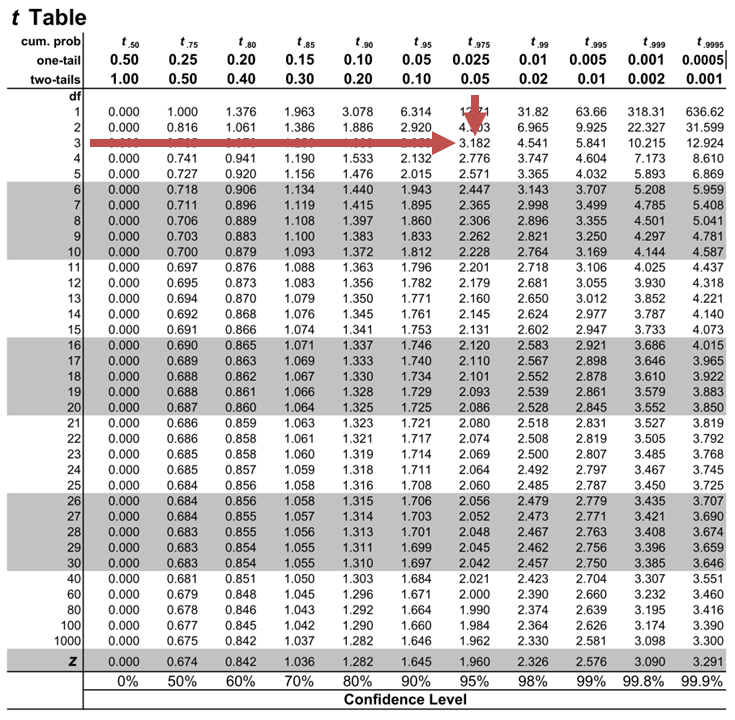# Hypothesis Testing in Regression Analysis

Hypothesis testing is used to confirm if the estimated regression coefficients bear any statistical significance.  Either the confidence interval approach or the t-test approach can be used in hypothesis testing. In this section, we will explore the t-test approach.

## The t-test Approach

The following are the steps followed in the performance of the t-test:

1. Set the significance level for the test.
2. Formulate the null and the alternative hypotheses.
3. Calculate the t-statistic using the formula below:

$$t=\frac{\widehat{b_1}-b_1}{s_{\widehat{b_1}}}$$

Where:

$$b_1$$ = True slope coefficient.

$$\widehat{b_1}$$ = Point estimate for $$b_1$$

$$b_1 s_{\widehat{b_1\ }}$$ = Standard error of the regression coefficient.

4. Compare the absolute value of the t-statistic to the critical t-value (t_c). Reject the null hypothesis if the absolute value of the t-statistic is greater than the critical t-value i.e., $$t\ >\ +\ t_{critical}\ or\ t\ <\ –t_{\text{critical}}$$.

#### Example: Hypothesis Testing of the Significance of Regression Coefficients

An analyst generates the following output from the regression analysis of inflation on unemployment:

$$\small{\begin{array}{llll}\hline{}& \textbf{Regression Statistics} &{}&{}\\ \hline{}& \text{Multiple R} & 0.8766 &{} \\ {}& \text{R Square} & 0.7684 &{} \\ {}& \text{Adjusted R Square} & 0.7394 & {}\\ {}& \text{Standard Error} & 0.0063 &{}\\ {}& \text{Observations} & 10 &{}\\ \hline {}& & & \\ \hline{} & \textbf{Coefficients} & \textbf{Standard Error} & \textbf{t-Stat}\\ \hline \text{Intercept} & 0.0710 & 0.0094 & 7.5160 \\\text{Forecast (Slope)} & -0.9041 & 0.1755 & -5.1516\\ \hline\end{array}}$$

At the 5% significant level, test the null hypothesis that the slope coefficient is significantly different from one, that is,

$$H_{0}: b_{1} = 1\ vs. \ H_{a}: b_{1}≠1$$

Solution

The calculated t-statistic, $$\text{t}=\frac{\widehat{b_{1}}-b_1}{\widehat{S_{b_{1}}}}$$ is equal to:

\begin{align*}\text{t}& = \frac{-0.9041-1}{0.1755}\\& = -10.85\end{align*}

The critical two-tail t-values from the table with $$n-2=8$$ degrees of freedom are:

$$\text{t}_{c}=±2.306$$Notice that $$|t|>t_{c}$$ i.e., ($$10.85>2.306$$)

Therefore, we reject the null hypothesis and conclude that the estimated slope coefficient is statistically different from one.

Note that we used the confidence interval approach and arrived at the same conclusion.

### Question

Neeth Shinu, CFA, is forecasting price elasticity of supply for a certain product. Shinu uses the quantity of the product supplied for the past 5months as the dependent variable and the price per unit of the product as the independent variable. The regression results are shown below.

$$\small{\begin{array}{lccccc}\hline \textbf{Regression Statistics} & & & & & \\ \hline \text{Multiple R} & 0.9971 & {}& {}&{}\\ \text{R Square} & 0.9941 & & & \\ \text{Adjusted R Square} & 0.9922 & & & & \\ \text{Standard Error} & 3.6515 & & & \\ \text{Observations} & 5 & & & \\ \hline {}& \textbf{Coefficients} & \textbf{Standard Error} & \textbf{t Stat} & \textbf{P-value}\\ \hline\text{Intercept} & -159 & 10.520 & (15.114) & 0.001\\ \text{Slope} & 0.26 & 0.012 & 22.517 & 0.000\\ \hline\end{array}}$$

Which of the following most likely reports the correct value of the t-statistic for the slope and most accurately evaluates its statistical significance with 95% confidence?

A. $$t=21.67$$; slope is significantly different from zero.

B. $$t= 3.18$$; slope is significantly different from zero.

C. $$t=22.57$$; slope is not significantly different from zero.

### Solution

The t-statistic is calculated using the formula:

$$\text{t}=\frac{\widehat{b_{1}}-b_1}{\widehat{S_{b_{1}}}}$$

Where:

• $$b_{1}$$ = True slope coefficient
• $$\widehat{b_{1}}$$ = Point estimator for $$b_{1}$$
• $$\widehat{S_{b_{1}}}$$ = Standard error of the regression coefficient

\begin{align*}\text{t}&=\frac{0.26-0}{0.012}\\&=21.67\end{align*}

The critical two-tail t-values from the t-table with $$n-2 = 3$$ degrees of freedom are:

$$t_{c}=±3.18$$Notice that $$|t|>t_{c}$$ (i.e $$21.67>3.18$$).

Therefore, the null hypothesis can be rejected. Further, we can conclude that the estimated slope coefficient is statistically different from zero.

Shop CFA® Exam Prep

Offered by AnalystPrepLevel I
Level II
Level III
All Three Levels
Featured Shop FRM® Exam PrepFRM Part I
FRM Part II
FRM Part I & Part II
Learn with Us

Subscribe to our newsletter and keep up with the latest and greatest tips for success
Shop Actuarial Exams PrepExam P (Probability)
Exam FM (Financial Mathematics)
Exams P & FM
Shop GMAT® Exam PrepComplete CourseSergio Torrico
2021-07-23
Excelente para el FRM 2 Escribo esta revisión en español para los hispanohablantes, soy de Bolivia, y utilicé AnalystPrep para dudas y consultas sobre mi preparación para el FRM nivel 2 (lo tomé una sola vez y aprobé muy bien), siempre tuve un soporte claro, directo y rápido, el material sale rápido cuando hay cambios en el temario de GARP, y los ejercicios y exámenes son muy útiles para practicar.diana
2021-07-17
So helpful. I have been using the videos to prepare for the CFA Level II exam. The videos signpost the reading contents, explain the concepts and provide additional context for specific concepts. The fun light-hearted analogies are also a welcome break to some very dry content. I usually watch the videos before going into more in-depth reading and they are a good way to avoid being overwhelmed by the sheer volume of content when you look at the readings.Kriti Dhawan
2021-07-16
A great curriculum provider. James sir explains the concept so well that rather than memorising it, you tend to intuitively understand and absorb them. Thank you ! Grateful I saw this at the right time for my CFA prep.nikhil kumar
2021-06-28
Very well explained and gives a great insight about topics in a very short time. Glad to have found Professor Forjan's lectures.Marwan
2021-06-22
Great support throughout the course by the team, did not feel neglectedBenjamin anonymous
2021-05-10
I loved using AnalystPrep for FRM. QBank is huge, videos are great. Would recommend to a friendDaniel Glyn
2021-03-24
I have finished my FRM1 thanks to AnalystPrep. And now using AnalystPrep for my FRM2 preparation. Professor Forjan is brilliant. He gives such good explanations and analogies. And more than anything makes learning fun. A big thank you to Analystprep and Professor Forjan. 5 stars all the way!michael walshe
2021-03-18
Professor James' videos are excellent for understanding the underlying theories behind financial engineering / financial analysis. The AnalystPrep videos were better than any of the others that I searched through on YouTube for providing a clear explanation of some concepts, such as Portfolio theory, CAPM, and Arbitrage Pricing theory. Watching these cleared up many of the unclarities I had in my head. Highly recommended.